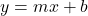Question

The slope of a line is 5. A (2, 1) is a point on the line. Another point, B, on the line has y=81. What is the x-value at this point, as denoted by d?

1.ngochoa
the x-value is 17
Step-by-step explanation:
slope = (y2 – y1) / (x2 – x1) = (81 – 1) / (x – 2) = 5
80 / (x – 1) = 5
x – 1 = 16
x = 17
hope this helps! <3

2.the x-value is 18
Step-by-step explanation:
Ok so the slope-intercept form can be represented as:where m=the slope, and b=y-intercept
we’re given the slope, so we can form the equation:, but we still don’t know the y-intercept.
We can solve for this using the point given, which we can substitute in known values:
substituting in 2 as x, and y as 1Multiply:Subtract 10 from both sidesNow we have the full equation:So, now we’re given another point, but this one is incomplete, we just have the y-value, so the point can be represented as (x, 81)
We can substitute in this known value to solve for x